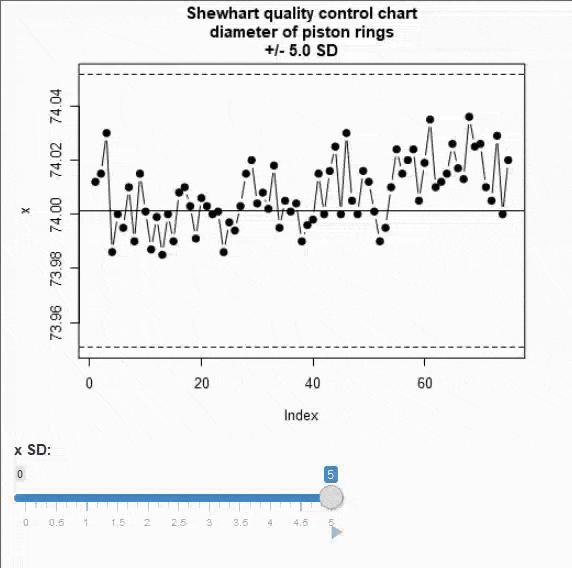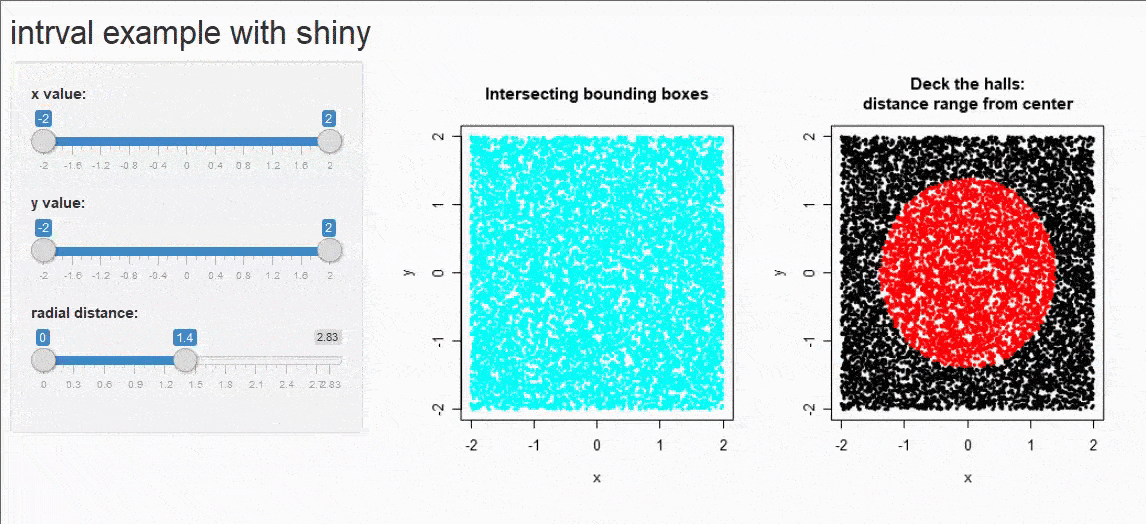[This article was first published on Peter Solymos - R related posts, and kindly contributed to R-bloggers]. (You can report issue about the content on this page here)
Want to share your content on R-bloggers? click here if you have a blog, or here if you don't.

The intrval R package is lightweight (~11K), standalone (apart from importing from graphics, has exactly 0 non-base dependency), and it has a very narrow scope: it implements relational operators for intervals — very well aligned with the tiny manifesto. In this post we will explore the use of the package in two shiny apps with sliders.

The first example uses a regular slider that returns a single value. To make that an interval, we will use standard deviation (SD, sigma) in a quality control chart (QCC). The code is based on the `pistonrings` data set from the qcc package. The Shewhart chart sets 3 sigma limit to indicate state of control. The slider is used to adjusts the sigma limit and the GIF below plays is as an animation.

```library(shiny)
library(intrval)
library(qcc)

data(pistonrings)
mu <- mean(pistonrings\$diameter[pistonrings\$trial])
SD <- sd(pistonrings\$diameter[pistonrings\$trial])
x <- pistonrings\$diameter[!pistonrings\$trial]

## UI function
ui <- fluidPage(
plotOutput("plot"),
sliderInput("x", "x SD:",
min=0, max=5, value=0, step=0.1,
animate=animationOptions(100)
)
)

# Server logic
server <- function(input, output) {
output\$plot <- renderPlot({
Main <- paste("Shewhart quality control chart",
"diameter of piston rings", sprintf("+/- %.1f SD", input\$x),
sep="\n")
iv <- mu + input\$x * c(-SD, SD)
plot(x, pch = 19, col = x %)(% iv +1, type = "b",
ylim = mu + 5 * c(-SD, SD), main = Main)
abline(h = mu)
abline(h = iv, lty = 2)
})
}

## Run shiny app
if (interactive()) shinyApp(ui, server)
```The second example uses range slider returning two values, which is our interval. To spice things up a bit, we combine intervals on two axes to color some random points. The next range slider defines a distance interval and colors the random points inside the ring.

```library(shiny)
library(intrval)

set.seed(1)
n <- 10^4
x <- round(runif(n, -2, 2), 2)
y <- round(runif(n, -2, 2), 2)
d <- round(sqrt(x^2 + y^2), 2)

## UI function
ui <- fluidPage(
titlePanel("intrval example with shiny"),
sidebarLayout(
sidebarPanel(
sliderInput("bb_x", "x value:",
min=min(x), max=max(x), value=range(x),
step=round(diff(range(x))/20, 1), animate=TRUE
),
sliderInput("bb_y", "y value:",
min = min(y), max = max(y), value = range(y),
step=round(diff(range(y))/20, 1), animate=TRUE
),
min = 0, max = max(d), value = c(0, max(d)/2),
step=round(max(d)/20, 1), animate=TRUE
)
),
mainPanel(
plotOutput("plot")
)
)
)

# Server logic
server <- function(input, output) {
output\$plot <- renderPlot({
iv1 <- x %[]% input\$bb_x & y %[]% input\$bb_y
iv2 <- x %[]% input\$bb_y & y %[]% input\$bb_x
iv3 <- d %()% input\$bb_d
op <- par(mfrow=c(1,2))
plot(x, y, pch = 19, cex = 0.25, col = iv1 + iv2 + 3,
main = "Intersecting bounding boxes")
plot(x, y, pch = 19, cex = 0.25, col = iv3 + 1,
main = "Deck the halls:\ndistance range from center")
par(op)
})
}

## Run shiny app
if (interactive()) shinyApp(ui, server)
```If you think there are other use cases for intrval in shiny applications, let me know in the comments section!